# How Does the ISEE Measure Mathematics Achievement?

How Does the ISEE Measure Mathematics Achievement?

* Numbers and operations

* Algebraic concepts

* Geometry

* Measurement

* Data analysis and probability

The mathematics achievement test consists of 47 questions, all of them of the standard multiple-choice type. Five of these questions are unscored.

The directions for the mathematics achievement test look similar to this:

Directions: Read each question and choose the best answer based on calculations in your head or in the margins of the test booklet.

Here are four sample ISEE mathematics achievement questions. Try each of these on your own before you read the explanation that accompanies it.

1. If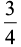of a class is absent and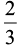of those present leave the room, what fraction of the original class remains in the room?

(A)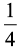(B)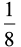(C)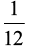(D)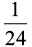The correct answer is (C). If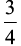are absent,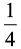are present. If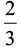of the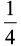present leave,of the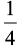remain.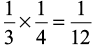remain in the room.

2. A cog wheel having 8 cogs plays into another cog wheel having 24 cogs. When the small wheel has made 42 revolutions, how many has the larger wheel made?

(A) 10

(B) 14

(C) 16

(D) 20

The correct answer is (B). The larger wheel is 3 times the size of the smaller wheel, so it makes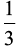the revolutions: 42 ÷ 3 = 14.

3. 75% of 4 is the same as what percent of 9?

(A) 25

(B) 33(C) 36

(D) 40

The correct answer is (B). 75% of 4 = 3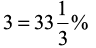of 9

4. If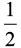cup of spinach contains 80 calories and the same amount of peas contains 300 calories, how many cups of spinach have the same caloric content as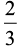cup of peas?

(A)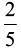(B) 1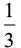(C) 2

(D) 2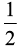The correct answer is (D).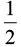cup spinach = 80 calories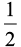cup peas = 300 calories

1 cup peas = 600 caloriescup peas = 400 calories

400 ÷ 80 = 5 half cups of spinach

= 2cups of spinach